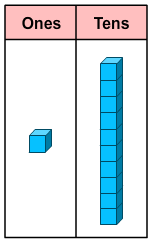# Count Tens and Ones Within 100

Rate 0 stars Common Core
Quiz size:
Message preview:
Someone you know has shared quiz with you:

To play this quiz, click on the link below:

https://www.turtlediary.com/quiz/count-tens-and-ones-within-100.html

Hope you have a good experience with this site and recommend to your friends too.

Login to rate activities and track progress.
Login to rate activities and track progress.

Remember the relation:

10 ones = 1 tenLet's consider an example. Let's write the tens and ones in the number 56.

56 = ___ ten(s) + ___ one(s)
We need to find the missing numbers in the above sentence.

We have:

56 = 50 + 6 = 5 tens + 6 ones

So, the missing numbers are 5 and 6.

ds

A B C D E F G H I J K L M N O P Q R S T U V W X Y Z

### Help

##### Remember :

The smallest number is the one that comes first while counting.

##### Solution :

To arrange the given numbers in order from smallest to greatest, find the smallest number among all the given numbers.

21,27,23

21 is the smallest number.